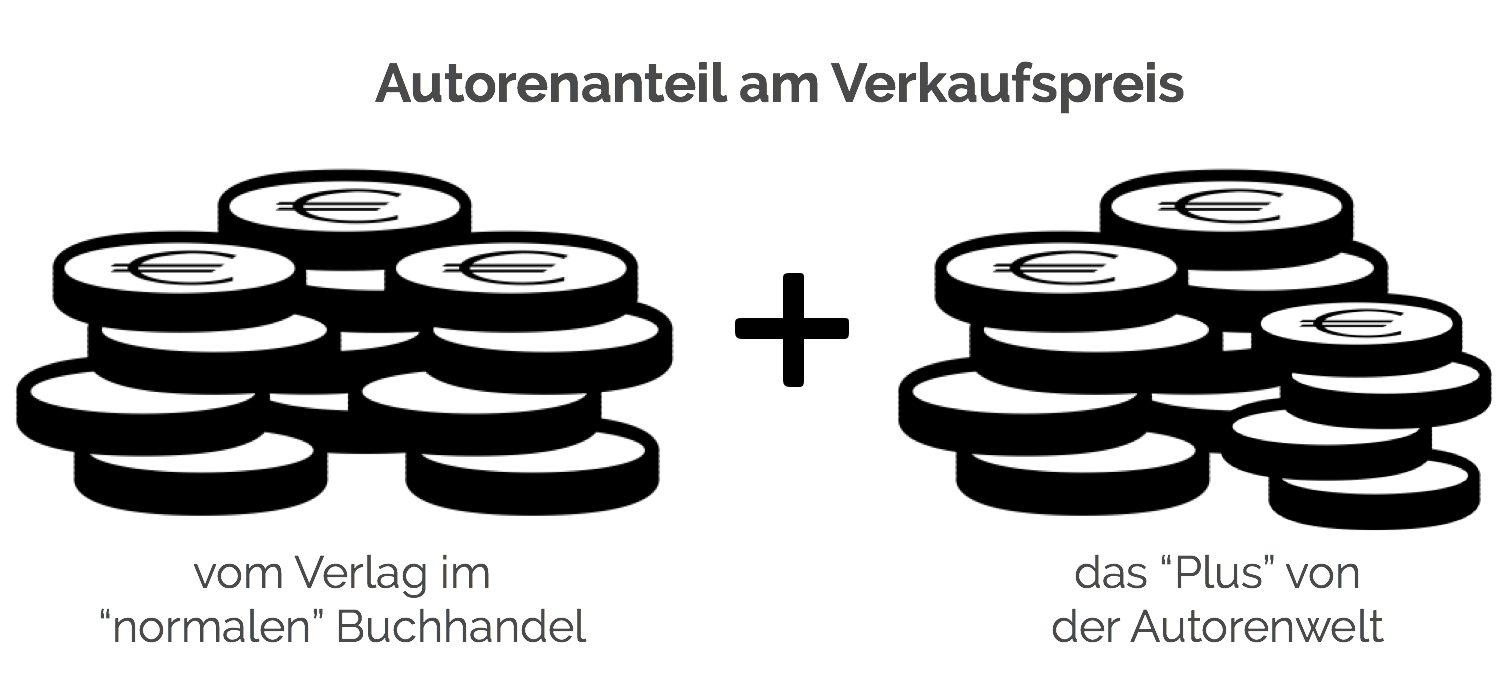Vector Bundle: Mathematics, Topology, Vector Space, Topological Space, Manifold, Algebraic Variety, Tangent Bundle, Fiber Bundle, Tangent Space, Hairy Ball Theorem, Parallelizable Manifold

Taschenbuch - 9786130354381
54,00 €
• Versandkostenfrei
• Hinweis: Print on Demand. Lieferbar innerhalb von 7 bis 10 Tagen
• inkl. MwSt. & Versandkosten (innerhalb Deutschlands)
• Hinweis: Print on Demand. Lieferbar innerhalb von 7 bis 10 Tagen
• inkl. MwSt. & Versandkosten (innerhalb Deutschlands)

Autorenfreundlich Bücher kaufen?!Beschreibung

High Quality Content by WIKIPEDIA articles! In mathematics, a vector bundle is a topological construction which makes precise the idea of a family of vector spaces parameterized by another space X (for example X could be a topological space, a manifold, or an algebraic variety): to every point x of the space X we associate (or 'attach') a vector space V(x) in such a way that these vector spaces fit together to form another space of the same kind as X (e.g. a topological space, manifold, or algebraic variety), which is then called a vector bundle over X. The simplest example is the case that the family of vector spaces is constant, i.e., there is a fixed vector space V such that V(x) = V for all x in X: in this case there is a copy of V for each x in X and these copies fit together to form the vector bundle X×V over X.

Details

 Verlag Betascript Publishers Ersterscheinung Februar 2010 Maße 220 mm x 150 mm x 12 mm Gewicht 302 Gramm Format Taschenbuch ISBN-13 9786130354381 Auflage Nicht bekannt Seiten 192

Schlagwörter

• american express
• apple pay
• bitcoin
• bitcoin cash
• ethereum